PHY.K02UF Molecular and Solid State Physics

## Crystal structure

In a crystal, atoms are arranged in straight rows in a three-dimensional periodic pattern. A small part of the crystal that can be repeated to form the entire crystal is called a unit cell.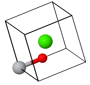Asymmetric unitPrimitive unit cell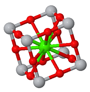Conventional unit cell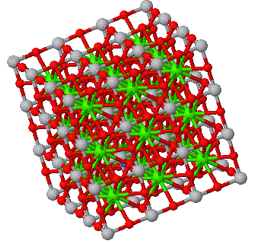Crystal

Devices such as solid state transistors, lasers, solar cells, and light emitting diodes are often made from single crystals. Many materials, including most metals and ceramics, are polycrystaline. This means there are many little crystals packed together where the orientation between the crystals is random. When the atoms of a material are not arranged in a regular pattern, it is called an amorphous material. An example of an amorphous material is glass. Even though not all solids are crystals, we will spend most of our time studying crystals since the translational symmetry makes them easier to decribe mathematically. Describing the behavior of more complicating materials usually builds on the understanding that has been acquired by studying crystals.

Some common crystal structures you should know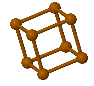Simple Cubic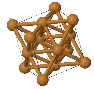Face Centered Cubic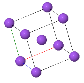Body Centered Cubic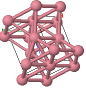Hexagonal Close Packed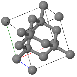Diamond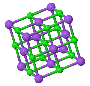NaCl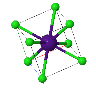CsCl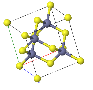ZincblendeWurzite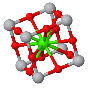Perovskite

• know what the primitive lattice vectors $(\vec{a}_1,\vec{a}_2,\vec{a}_3)$ are and how they can be used to calculate the volume of a primitive unit cell.
• The volume of a unit cell is $\vec{a}_1\cdot(\vec{a}_2\times\vec{a}_3)$.
• A translation vector of the crystal is $\vec{T}=l\vec{a}_1+m\vec{a}_2+n\vec{a}_3$ where $l,m,n$ are integers.